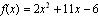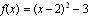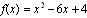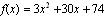Java Games: Flashcards, matching, concentration, and word search.# Precalc 2.1 to 2.2 review

review graphing quadratics and polynomials

AB
x and y intercepts of,y = -6, x = 0.5, x = -6
x and y intercepts of,y = 1, x = 2 +/- sqrt 3
x and y intercepts of,y = 0, x = 0, 1, -2
vertex of,(0, -4)
vertex of,(3, -5)
vertex of,(-5, -1)
parabola with vertex (-3, 3) passing through point (-2, 1)y=-2(x+3)^2+3
parabola with vertex (2, 0) passing through point (0, 4)y=(x - 2)^2
polynomial with zeros x = 0, 1, 2, 3y = x^4 - 6x^3 + 11x^2 - 6x
polynomial with zeros. x = 2/3, x = -5y = 3x^2 + 13x - 10
polynomial with zeros x = 1, 1, 3y = x^3 - 5x^2 + 7x - 3
standard form of y = x^2 + 4x + 7y = (x + 2)^2 + 3
standard form of y = -x^2 - 4x + 1y = - (x + 2)^2 + 5
left and right behavior of y = 4x^2 - 2x + 1up left, up right
left and right behavior of y = 3x^5 + 7x + 2down left, up right
left and right behavior of y = - 2x^5 + 2x^2 - 1up left, down right
left and right behavior of y = - 2x^4 + 3x^3 + 5x^2down left, down right
Find all real zeros of y = x^3 - 3x^2 - 4xx = 0, 4, -1
find all real zeros of y = x^6 - x^2x = 0, 1, -1
find all real zeros of y = x^3 + xx = 0
find all real zeros of y = x^4 - 5x^2 - 36x = 3, -3
find all real zeros of y = x^3+ 3x^2- 16x - 48x = -3, 4, -4
find all real zeros of y = 9x^4 - 37x^2 + 4x = 1/3, - 1/3, 2, -2Mrs. Miller math teacher Susquenita High School Duncannon, PA

 This activity was created by a Quia Web subscriber. Learn more about QuiaCreate your own activities